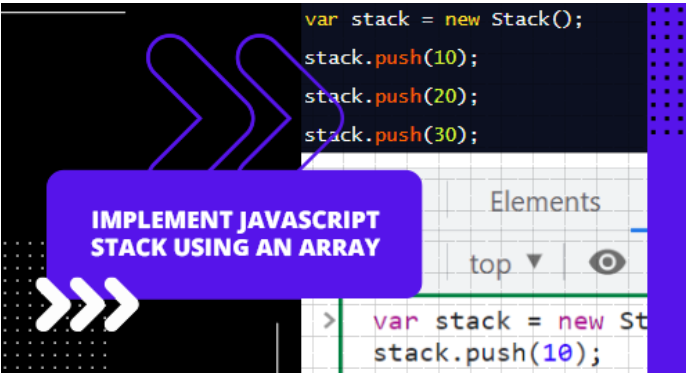/  Technology   /  Implementing JavaScript Stack Using Array## Implementing JavaScript Stack Using Array

The Last In First Out (LIFO) principle describes a data structure in which elements are placed in Last In First Out order. In general, the LIFO principle refers to the arrangement of elements in such a way that the most recent element is removed first, and the oldest element is removed last. The arrangement of the elements in Stack can be understood as the arrangement of dishing plates in which the plate which was kept first will be used at the end. LIFO refers to such an arrangement.

Stacks are composed of two main functions, push() and pop(). Stack performs both of these operations at the top of the stack. The push() operation inserts/adds elements to the stack, whereas the pop() operation removes/pops elements from the stack. Since elements are always pushed and popped out of a stack from the top, push() and pop() occur at the top.

## Operations on Stack

• push(): Push() is used to add elements to the stack.
• pop(): Stack elements can be removed using the pop() operation.
• peek(): Using the peek() operation, we can obtain the top element of the stack.
• length(): A stack’s length can be determined by using the length() operation.
• search(): A search() operation is used for searching elements within a stack, regardless of whether they are present.
• print(): Stack elements are printed using the print() operation.
• isEmpty(): IsEmpty() checks whether the stack is empty.

### push() operation

The stack method is used to add elements to the top of the list.

Below is an example of how to use the push() method:

```stackpushmethod (e) {
this.ele[this.top] = e;
thisthis.top = this.top + 1;
}  ```
• In our stackpushmethod() function, we have passed an argument named e. This argument contains the value that will be inserted into the stack.
• With this function, we have accessed the value of e in the array ele and at the top of the array.
• Due to the fact that the top variable must point to the next empty array index in the stack, the value of top is increased by 1.

### pop() operation

For removing or deleting elements from the top position of a stack, the pop() method is used.

Below is an example of a stackpop() operation:

```stackpopmethod () {
thisthis.top = this.top - 1;
return this.data.pop ();
}  ```
• We have created a stackpopmethod () function, under which the first step is to decrease the value of top by one. As a result, the top variable must point to the position of the previous element.
• This operator will be used in the next step to pop out the value at the top of the stack.

### length () operation

Utilizing the top variable, the length () operation of the Stack returns the stack’s length.

The following example illustrates how to use the length () operation:

```stacklengthmethod () {
return this.top;
}  ```

We have created a stacklengthmethod () function that calculates the stack’s length from the top.

### peek () operation

This operation retrieves/fetches the value at the top of the stack.

```peekmethod() {
return this.data[this.top -1 ];
}  ```
• The peekmethod () function returns the element present at the top of the stack in the example above.
• We have used top – 1 as the top variable points to the top position in the stack.

### print () operation

Printing the elements present in the stack is accomplished using the print () operation. As a result, it is similar to the printf function in C programming.

The following example illustrates how print () is implemented:

```function printmethod() {
var i = this.top - 2; // as top variable points to the element position
while(i >= 0) {
console.log(this.data[i]);
i--;
}
}  ```
• We have created a function printmethod () that initializes a variable t with the value top – 2.
• In order to print all the values of the stack from top to bottom, a while loop is used.
• From the last to the top, i.e., up to the 0th, the loop will begin
• As per the index value, each array index value will be printed.
• Finally, the value is decremented as i–.